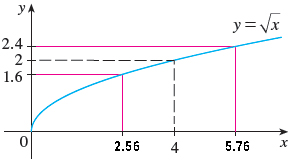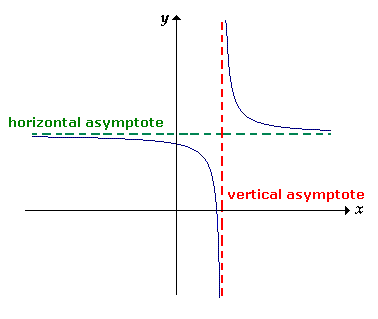# Limits and Continuity

By Suresh Chand

Let f(x) be a function defined on some open interval that contains the number a except possibly at itself. then we say that the limit of f(x) on x approaches a is L, and we write

$$\lim_{x \to a} f(x) = L$$

If for every number ε > 0 there is a number δ > 0 such that if 0 < |x – a| < δ then |f(x) – L| < ε.

Example 3: Use the graph of f(x) = $$\sqrt{x}$$ to find a number δ such that if |x – 4| < δ then $$|\sqrt{x}-2| < 0.4$$.Solution:

Here limit of the function f(x) = $$\sqrt{x}$$ at x = 4 is 2 i.e. L = 2 and a = 4, ε = 0.4

Now

-0.4 < $$\sqrt{x}$$ – 2 < 0.4

1.6 < $$\sqrt{x}$$ < 2.4 whenever |x – 4| < δ

We need to concentrate near region of the point (4,2)

We need to find the values of x for which the curve y = $$\sqrt{x}$$ lies between the horizontal line y= 1.6 and y = 2.4. Therefore, we graph the curve y = $$\sqrt{x}$$, y = 1.6 and y = 2.4 near point (4,2).

Now the point of intersection of y = $$\sqrt{x}$$ and  y  =1.6 is x = 2.56

Again the point of intersection of y = $$\sqrt{x}$$ and y = 2.4 is x = 5.76

Now rounding to be safe we have if 2.56 < x < 5.76 then 1.6 < $$\sqrt{x}$$ < 2.4

This interval (2.56, 5.76) is not symmetric about x = 4. The distance from x = 4 to the left end point is 4 – 2.56 = 1.44 and the distance of x = 4 from the right end point.

x = 5.76 is 5.76 – 4  = 1.76

Therefore, δ = smallest of two numbers  = 1.44

Hence we can write |x – 4| < 1.44 then |$$\sqrt{x}$$ – 2| < 0.4

## Continuity

Definition: A function f(x) is continuous at x = a if $$\lim_{x \to a} f(x) = f(a)$$ [Functional Value  = Limiting Value]

Precisely,  a function f(x) is continuous at x = a if

1. f(a) is defined (i.e. a is in the domain of f)
2. $$\lim_{x \to a} f(x)$$ exists
3. $$\lim_{x \to a} f(x) = f(a)$$

Example: Use Continuity to evaluate limit.

$$\lim_{x \to 4} \frac{5 + \sqrt{x}}{\sqrt{5 + x}}$$

Solution:

Since the function $$f(x) = \frac{g(x)}{h(x)}$$ is being a quotient of two continuous functions g(x) = 5 + $$\sqrt{x}$$ and h(x) = $$\sqrt{5 + x}$$ everywhere in their domain. In particular at x = 4 and hence the quotient function f(x) is also continuous at x = 4

$$\lim_{x \to 4}f(x) = f(4)$$ $$\frac{5 + \sqrt{4}}{\sqrt{5 + 4}} = \frac{7}{3}$$

### Horizontal Asymptotes

The line y = L is called a horizonal asymptotes of the curve y = f(x) if either

$$\lim_{x \to ∞} f(x) = L \enspace or \enspace \lim_{x \to -∞} f(x) = L$$Example: Find the horizontal asymptotes of curve y = tan-1x

Solution:

Now

$$\lim_{x \to ∞} tan^{-1}x = tan^{-1}(∞) = \frac{\pi}{2}$$

Therefore, y = $$\frac{\pi}{2}$$ is a horizontal asymptotes

Again

$$\lim_{x \to -∞} tan^{-1}x = tan^{-1}(-∞) = -\frac{\pi}{2}$$

which shows that y = –$$\frac{\pi}{2}$$ is another horizontal asymptotes of same function y = tan-1x.

### Vertical Asymptotes

Let f(x) be a given function. If there exists a number a such that any one of the following is true

1. $$\lim_{x \to a} f(x) = ∞ \enspace or \enspace -∞$$
2. $$\lim_{x \to a^{-}} f(x) = ∞ \enspace or \enspace -∞$$
3. $$\lim_{x \to a^{+}} f(x) = ∞ \enspace or \enspace -∞$$

Then x = 0 is called the vertical asymptotes.Example: Find the vertical Asymptotes of $$y = \frac{x + 2}{x^2 + 2x – 8}$$.

Solution:

We will get the y = ∞ when the denominator part is zero. So,

x2 + 2x – 8 = 0

(x + 4)(x – 2) = 0

x = –4 or x = 2

So, y = -4 and x = 2 are the vertical asymptotes.

Important Questions